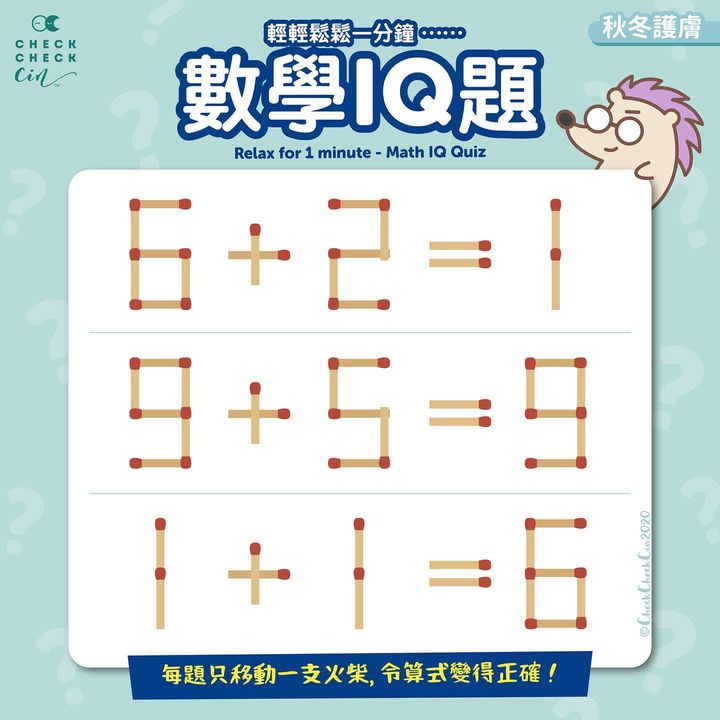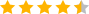December 5, 2020

【輕輕鬆鬆一分鐘⋯⋯數學IQ題】

⭐都是很簡單的加數啊
⭐動動腦筋不易老
#星期日輕輕鬆鬆一分鐘

1. 6 + 2 = 1
2. 9 + 5 = 9
3. 1 + 1 = 6

（答案於留言欄公佈）

Relax for 1 minute – Math IQ Quiz

In the picture below, you can only move one matchstick in each question to make sure the equations are correct.

1. 6 + 2 = 1
2. 9 + 5 = 9
3. 1 + 1 = 6